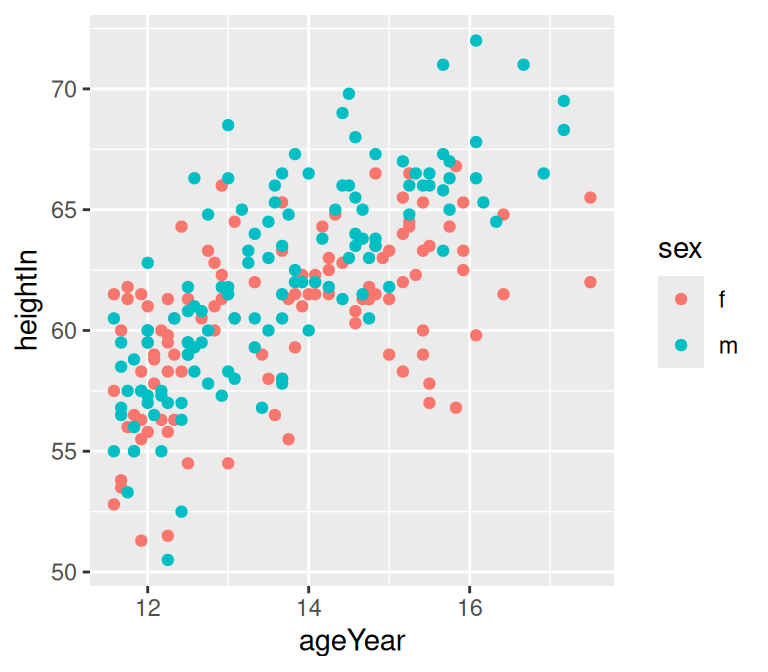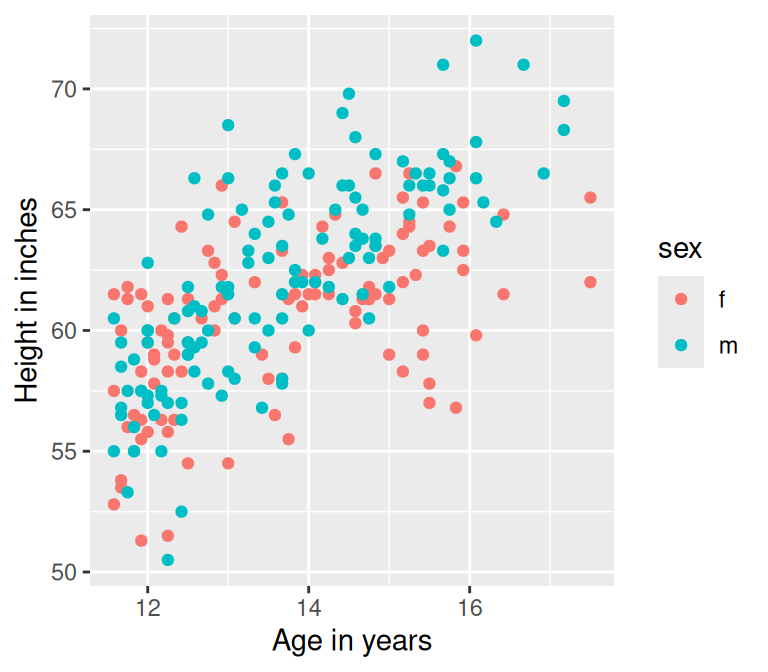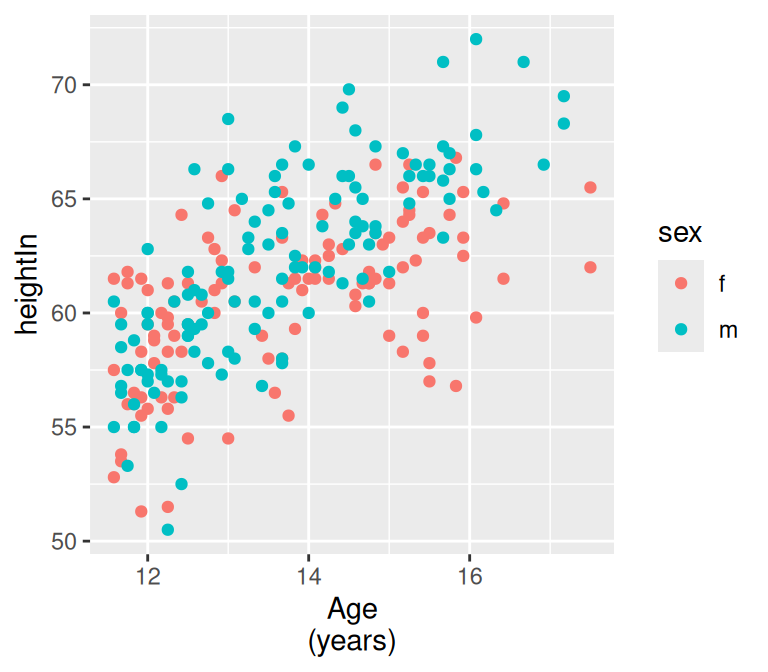## 8.10 Changing the Text of Axis Labels

### 8.10.1 Problem

You want to change the text of axis labels.

### 8.10.2 Solution

Use `xlab()` or `ylab()` to change the text of the axis labels (Figure 8.19):

``````library(gcookbook)  # Load gcookbook for the heightweight data set

hw_plot <- ggplot(heightweight, aes(x = ageYear, y = heightIn, colour = sex)) +
geom_point()

# With default axis labels
hw_plot

# Set the axis labels
hw_plot +
xlab("Age in years") +
ylab("Height in inches")``````Figure 8.19: Scatter plot with the default axis labels (left); Manually specified labels for the x- and y-axes (right)

### 8.10.3 Discussion

By default the graphs will just use the column names from the data frame as axis labels. This might be fine for exploring data, but for presenting it, you may want more descriptive axis labels.

Instead of `xlab()` and `ylab()`, you can use `labs()`:

``````hw_plot +
labs(x = "Age in years", y = "Height in inches")``````

Another way of setting the axis labels is in the scale specification, like this:

``````hw_plot +
scale_x_continuous(name = "Age in years")``````

This may look a bit awkward, but it can be useful if you’re also setting other properties of the scale, such as the tick mark placement, range, and so on.

This also applies, of course, to other axis scales, such as `scale_y_continuous()`, `scale_x_discrete()`, and so on.

You can also add line breaks with `\n`, as shown in Figure 8.20:

``````hw_plot +
scale_x_continuous(name = "Age\n(years)")``````Figure 8.20: X-axis label with a line break